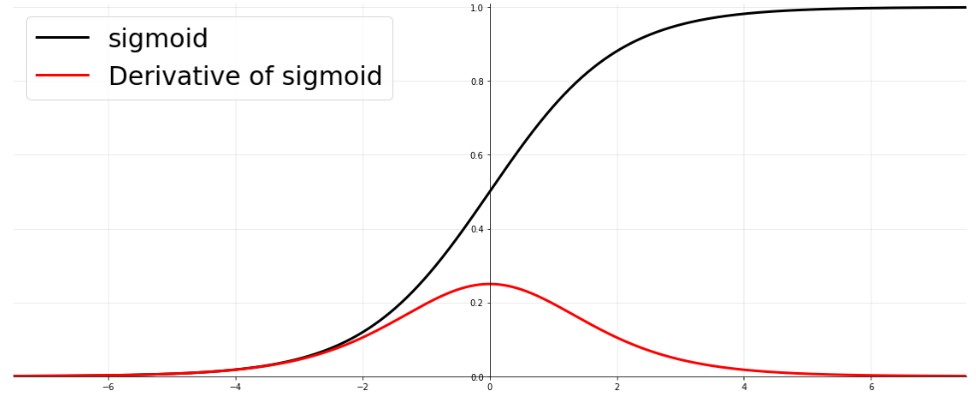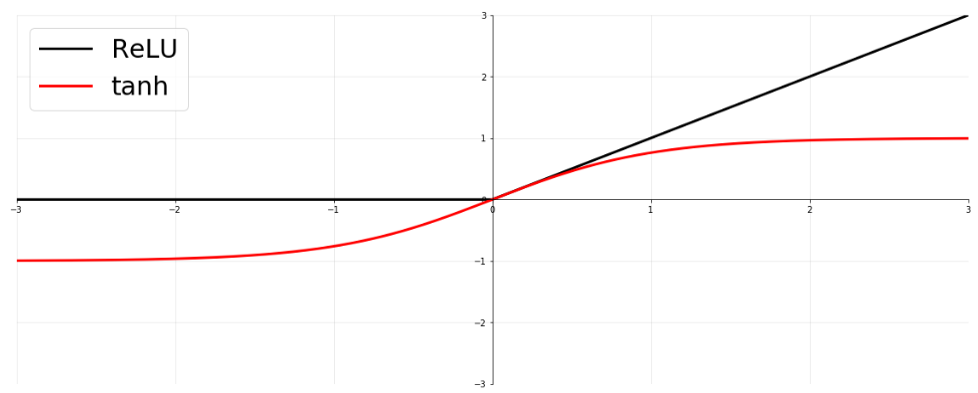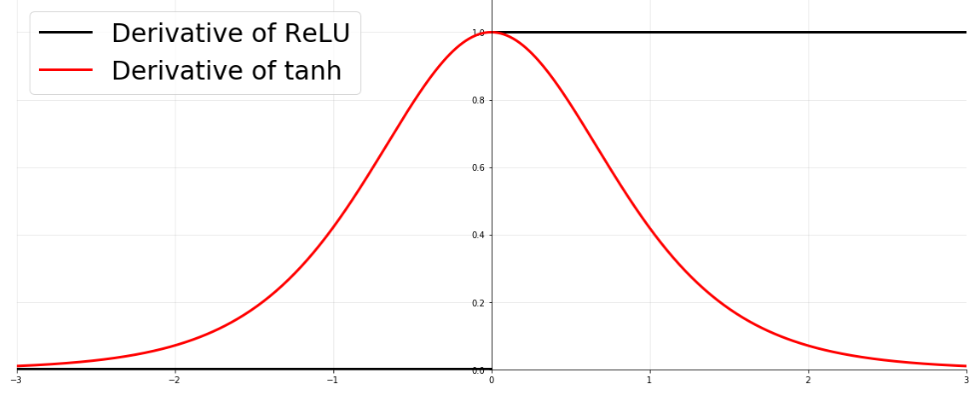By Prof. Seungchul Lee
http://iai.postech.ac.kr/
Industrial AI Lab at POSTECH

# 1. Nonlinear Activation Function¶

• As more layers using certain activation functions are added to neural networks, the gradients of the loss function approaches zero, making the network hard to train.

• For example,

$$\frac{z}{u} = \frac{z}{y} \cdot \frac{y}{x} \cdot \frac{x}{\omega} \cdot \frac{\omega}{u}$$• Rectifiers
• The use of the ReLU activation function was a great improvement compared to the historical tanh.• This can be explained by the derivative of ReLU itself not vanishing, and by the resulting coding being sparse (Glorot et al., 2011).# 2. Batch Normalization¶

Batch normalization is a technique for improving the performance and stability of artificial neural networks.

It is used to normalize the input layer by adjusting and scaling the activations.